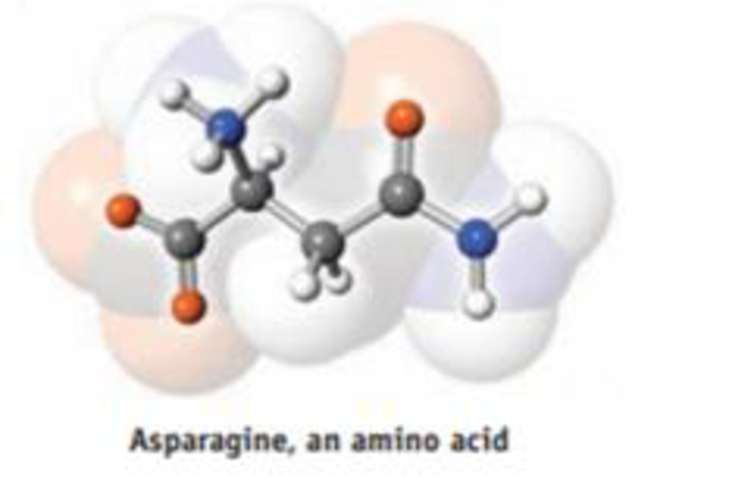Chapter 2, Problem 34PS

Chapter
Section
Textbook Problem

A model of the amino acid asparagine is illustrated here. Write the molecular formula for the compound, and draw its structural formula.Interpretation Introduction

Interpretation: For the given molecular model of asparagine, the molecular formula, and structural formula are needed to be determined.

Concept introduction:

• Molecular formula of compound is the representation with symbols of each atom and their numbers present in the compound.
• Structural formula of compound is the representation of all the atoms and their respective bonding in a plane.
Explanation

In the given model of asparagine, there are four Carbon atoms, eight Hydrogen atoms, 2 Nitrogen atom, and 3 Oxygen atoms.

Molecular formula of compound is the representation with symbols of each atom and their numbers present in the compound.

Therefore,

The molecular formula of asparagine is C4H8

Still sussing out bartleby?

Check out a sample textbook solution.

See a sample solution

The Solution to Your Study Problems

Bartleby provides explanations to thousands of textbook problems written by our experts, many with advanced degrees!

Get Started